Answer all questions and submit them either as an IPython notebook, LaTeX document, or Markdown document. Provide full answers for each question, including interpretation of the results. Each question is worth 25 points.

This homework is due on Monday, December 8, 2016.

In :
%matplotlib inline
import numpy as np
# import pymc as pm
import pylab as plt
import pandas as pd

# Set seed
np.random.seed(42)


Question 1¶

The titanic.xls spreadsheet in the data directory contains data regarding the passengers on the Titanic when it sank in 1912. A recent Kaggle competition was based on predicting survival for passengers based on the attributes in the passenger list.

Use scikit-learn to build both a support vector classifier and a logistic regression model to predict survival on the Titanic. Use cross-validation to assess your models, and try to tune them to improve performance.

Discuss the benefits and drawbacks of both approaches for application to such problems.

In :
titanic = pd.read_excel('/Users/fonnescj/Teaching/Bios8366/data/titanic.xls', 'titanic')

Out:
pclass survived name sex age sibsp parch ticket fare cabin embarked boat body home.dest
0 1 1 Allen, Miss. Elisabeth Walton female 29.0000 0 0 24160 211.3375 B5 S 2 NaN St Louis, MO
1 1 1 Allison, Master. Hudson Trevor male 0.9167 1 2 113781 151.5500 C22 C26 S 11 NaN Montreal, PQ / Chesterville, ON
2 1 0 Allison, Miss. Helen Loraine female 2.0000 1 2 113781 151.5500 C22 C26 S NaN NaN Montreal, PQ / Chesterville, ON
3 1 0 Allison, Mr. Hudson Joshua Creighton male 30.0000 1 2 113781 151.5500 C22 C26 S NaN 135.0 Montreal, PQ / Chesterville, ON
4 1 0 Allison, Mrs. Hudson J C (Bessie Waldo Daniels) female 25.0000 1 2 113781 151.5500 C22 C26 S NaN NaN Montreal, PQ / Chesterville, ON
In :
titanic_data = titanic.copy()[['pclass', 'survived', 'sex', 'age', 'sibsp', 'parch', 'fare', 'embarked']]

titanic_data['female'] = titanic_data.sex.replace(to_replace= ['male', 'female'], value= [0, 1], regex= False)
titanic_data = titanic_data.drop('sex', axis=1)

In :
titanic_data.embarked.value_counts()

Out:
S    914
C    270
Q    123
Name: embarked, dtype: int64
In :
titanic_data.embarked = titanic_data.embarked.replace({'S':0, 'C':1, 'Q':2})

In :
titanic_data = titanic_data.dropna(subset=['embarked'])

In :
from sklearn import preprocessing

lb = preprocessing.LabelBinarizer()
lb.fit(list(titanic_data.embarked.dropna()))
embarked_classes = lb.transform(titanic_data.embarked.values)

titanic_data['C'] = embarked_classes.T
titanic_data['Q'] = embarked_classes.T
titanic_data = titanic_data.drop('embarked', axis=1)

In :
X = titanic_data.dropna()
y = X.pop('survived')

In :
from sklearn.model_selection import train_test_split, GridSearchCV
from sklearn.preprocessing import normalize

X_normalized = normalize(X.values.astype(float))

X_train, X_test, y_train, y_test = train_test_split(X_normalized, y)

In :
from sklearn import svm

svc1 = svm.SVC(kernel='linear')
svc1.fit(X_train, y_train)

svc1.score(X_train, y_train)

Out:
0.6662404092071611
In :
svc1.score(X_test, y_test)

Out:
0.65900383141762453
In :
parameters = {'kernel':('linear', 'rbf', 'poly'), 'C':[0.1, 0.5, 1, 5, 10]}

np.random.seed(3535)
gscv1 = GridSearchCV(svm.SVC(), parameters, cv= 5) # default is 3-fold
gscv1.fit(X_normalized, y)

Out:
GridSearchCV(cv=5, error_score='raise',
estimator=SVC(C=1.0, cache_size=200, class_weight=None, coef0=0.0,
decision_function_shape=None, degree=3, gamma='auto', kernel='rbf',
max_iter=-1, probability=False, random_state=None, shrinking=True,
tol=0.001, verbose=False),
fit_params={}, iid=True, n_jobs=1,
param_grid={'kernel': ('linear', 'rbf', 'poly'), 'C': [0.1, 0.5, 1, 5, 10]},
pre_dispatch='2*n_jobs', refit=True, return_train_score=True,
scoring=None, verbose=0)
In :
gscv1.best_estimator_

Out:
SVC(C=10, cache_size=200, class_weight=None, coef0=0.0,
decision_function_shape=None, degree=3, gamma='auto', kernel='linear',
max_iter=-1, probability=False, random_state=None, shrinking=True,
tol=0.001, verbose=False)
In :
gscv1.best_score_

Out:
0.68935762224352826
In :
gscv1.score(X_test, y_test)

Out:
0.73946360153256707
In :
from sklearn.linear_model import LogisticRegression

lrmod = LogisticRegression(C= 10000)  # low penalty
lrmod.fit(X_train, y_train)

Out:
LogisticRegression(C=10000, class_weight=None, dual=False, fit_intercept=True,
intercept_scaling=1, max_iter=100, multi_class='ovr', n_jobs=1,
penalty='l2', random_state=None, solver='liblinear', tol=0.0001,
verbose=0, warm_start=False)
In :
lrmod.score(X_train, y_train)

Out:
0.76086956521739135
In :
lrmod.score(X_test, y_test)

Out:
0.77394636015325668
In :
parameters2 = {'penalty':('l1', 'l2'), 'C':[0.0001, 0.001, 0.01, 0.1, 1, 10, 100, 1000, 10000],
'intercept_scaling':[1, 10, 50, 100, 500, 1000]}  # increase intercept_scaling to minimize the penalty on the intercept

np.random.seed(3535)
gscv2 = GridSearchCV(LogisticRegression(), parameters2, cv= 5) # default is 3-fold
gscv2.fit(X_normalized, y)

Out:
GridSearchCV(cv=5, error_score='raise',
estimator=LogisticRegression(C=1.0, class_weight=None, dual=False, fit_intercept=True,
intercept_scaling=1, max_iter=100, multi_class='ovr', n_jobs=1,
penalty='l2', random_state=None, solver='liblinear', tol=0.0001,
verbose=0, warm_start=False),
fit_params={}, iid=True, n_jobs=1,
param_grid={'intercept_scaling': [1, 10, 50, 100, 500, 1000], 'C': [0.0001, 0.001, 0.01, 0.1, 1, 10, 100, 1000, 10000], 'penalty': ('l1', 'l2')},
pre_dispatch='2*n_jobs', refit=True, return_train_score=True,
scoring=None, verbose=0)
In :
gscv2.best_estimator_

Out:
LogisticRegression(C=100, class_weight=None, dual=False, fit_intercept=True,
intercept_scaling=500, max_iter=100, multi_class='ovr', n_jobs=1,
penalty='l1', random_state=None, solver='liblinear', tol=0.0001,
verbose=0, warm_start=False)
In :
gscv2.best_score_

Out:
0.73825503355704702
In :
gscv2.score(X_test, y_test)

Out:
0.77777777777777779

Logistic regression performed slightly better, and is more easily interpretable, as well as being less computationally intensive.

Question 2¶

The file TNNASHVI.txt in your data directory contains daily temperature readings for Nashville, courtesy of the Average Daily Temperature Archive. This data, as one would expect, oscillates annually. Using either GPy or PyMC, use a Gaussian process to fit a non-parametric regression model to this data, choosing an appropriate covariance function. Plot 10 regression lines drawn from your process.

In :
%matplotlib inline
import pandas as pd

names=['month','day','year','temp'], na_values=-99)

In :
daily_temps.head()

Out:
month day year temp
0 1 1 1995 46.7
1 1 2 1995 28.7
2 1 3 1995 27.9
3 1 4 1995 27.0
4 1 5 1995 18.5
In :
y = daily_temps.temp.dropna().values[:1000]
N = len(y)
x = np.arange(N)/1000

In :
plt.plot(x, daily_temps.temp[:1000].values, 'b.')

Out:
[<matplotlib.lines.Line2D at 0x14259e0b8>]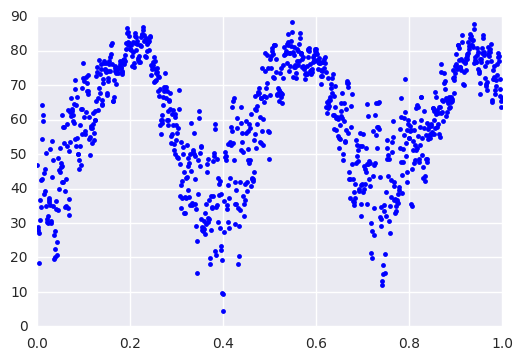In :
import GPy

In :
kernel = GPy.kern.PeriodicMatern32(1, variance=10, lengthscale=1.2)

In :
X = x.reshape(-1, 1)
Y = y.reshape(-1, 1)

In :
K_xx = kernel.K(X,X)
plt.imshow(np.asmatrix(K_xx), cmap='terrain', interpolation='nearest')
plt.axis('off');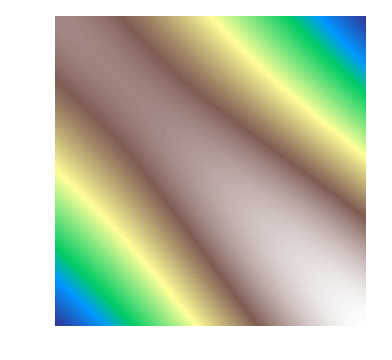In :
m = GPy.models.GPRegression(X=X, Y=Y, kernel=kernel)
m.optimize()

In :
m.plot()
plt.xlim(0, 1)
plt.ylim(0,120);

 /Users/fonnescj/anaconda3/envs/bios8366/lib/python3.5/site-packages/matplotlib/figure.py:1742: UserWarning:This figure includes Axes that are not compatible with tight_layout, so its results might be incorrect.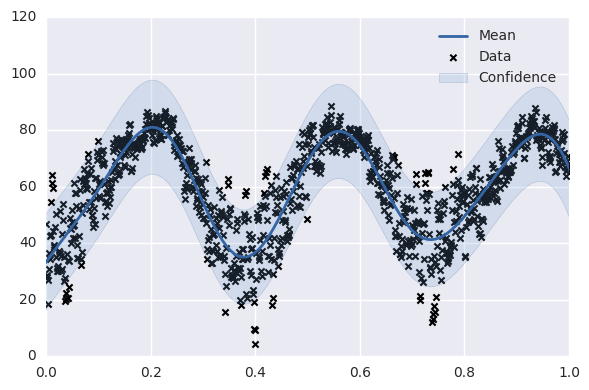In :
draws = m.posterior_samples(X, full_cov=True, size=10)

 /Users/fonnescj/anaconda3/envs/bios8366/lib/python3.5/site-packages/GPy/core/gp.py:492: RuntimeWarning:covariance is not positive-semidefinite.

In :
y_sim, MSE = m.predict(X)

In :
plt.plot(x, draws, color='SeaGreen', alpha=0.1)
plt.plot(x, y_sim - 2*MSE ** 0.5, 'SeaGreen')
plt.plot(x, y_sim + 2*MSE ** 0.5, 'SeaGreen')

plt.xlim(0, 1)
plt.ylim(0,120);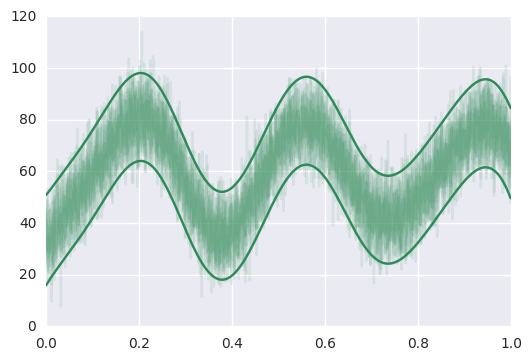Question 3¶

The data in prostate.data.txt come from a study by Stamey et al. (1989), which examined the correlation between the level of prostate-specific antigen (lpsa) and a number of clinical measures in men who were about to receive a radical prostatectomy. The variables are log cancer volume (lcavol), log prostate weight (lweight), age, log of the amount of benign prostatic hyperplasia (lbph), seminal vesicle invasion (svi), log of capsular penetration (lcp), Gleason score (gleason), and percent of Gleason scores 4 or 5 (pgg45).

1. Select (your choice) five competing 3-variable linear regression models, and compare them using AIC, five-fold and ten-fold cross-validation. Discuss the results.

2. An alternative method for model assessment is to fit the models on a set of bootstrap samples, and then keep track of how well it predicts the original training set. If $\hat{f}^b(x_i)$ is the predicted value at $x_i$, from the model fitted to the bth bootstrap dataset, such an estimate is: $$\frac{1}{B} \frac{1}{N} \sum_{b=1}^B \sum_{i=1}^N L(y_i,\hat{f}^b(x_i))$$ However, because the bootstrap samples tend to contain many observations in common among the set of bootstrap samples, this estimate will tend to underestimate the true error rate. The so-called .632 estimator aleviates this bias by returning a weighted average of the training error (average loss over the training sample) and the leave-one-out (LOO) bootstrap error: $$\hat{err}^{(.632)} = 0.368 \, \bar{err} + 0.632 \, \hat{err}^{(1)}$$ where: $$\bar{err} = \frac{1}{N}\sum_{i=1}^N L(y_i, \hat{f}(x_i))$$ Repeat the assesment from part (1) using the .632 estimator, and compare the result to the other approaches.

In :
# Part 1

Out:
lcavol lweight age lbph svi lcp gleason pgg45 lpsa train
1 -0.579818 2.769459 50 -1.386294 0 -1.386294 6 0 -0.430783 T
2 -0.994252 3.319626 58 -1.386294 0 -1.386294 6 0 -0.162519 T
3 -0.510826 2.691243 74 -1.386294 0 -1.386294 7 20 -0.162519 T
4 -1.203973 3.282789 58 -1.386294 0 -1.386294 6 0 -0.162519 T
5 0.751416 3.432373 62 -1.386294 0 -1.386294 6 0 0.371564 T
In :
prostate.describe()

Out:
lcavol lweight age lbph svi lcp gleason pgg45 lpsa
count 97.000000 97.000000 97.000000 97.000000 97.000000 97.000000 97.000000 97.000000 97.000000
mean 1.350010 3.628943 63.865979 0.100356 0.216495 -0.179366 6.752577 24.381443 2.478387
std 1.178625 0.428411 7.445117 1.450807 0.413995 1.398250 0.722134 28.204035 1.154329
min -1.347074 2.374906 41.000000 -1.386294 0.000000 -1.386294 6.000000 0.000000 -0.430783
25% 0.512824 3.375880 60.000000 -1.386294 0.000000 -1.386294 6.000000 0.000000 1.731656
50% 1.446919 3.623007 65.000000 0.300105 0.000000 -0.798508 7.000000 15.000000 2.591516
75% 2.127041 3.876396 68.000000 1.558145 0.000000 1.178655 7.000000 40.000000 3.056357
max 3.821004 4.780383 79.000000 2.326302 1.000000 2.904165 9.000000 100.000000 5.582932
In :
pd.scatter_matrix(prostate, alpha=0.8, figsize=(12, 12), diagonal="kde", grid=False);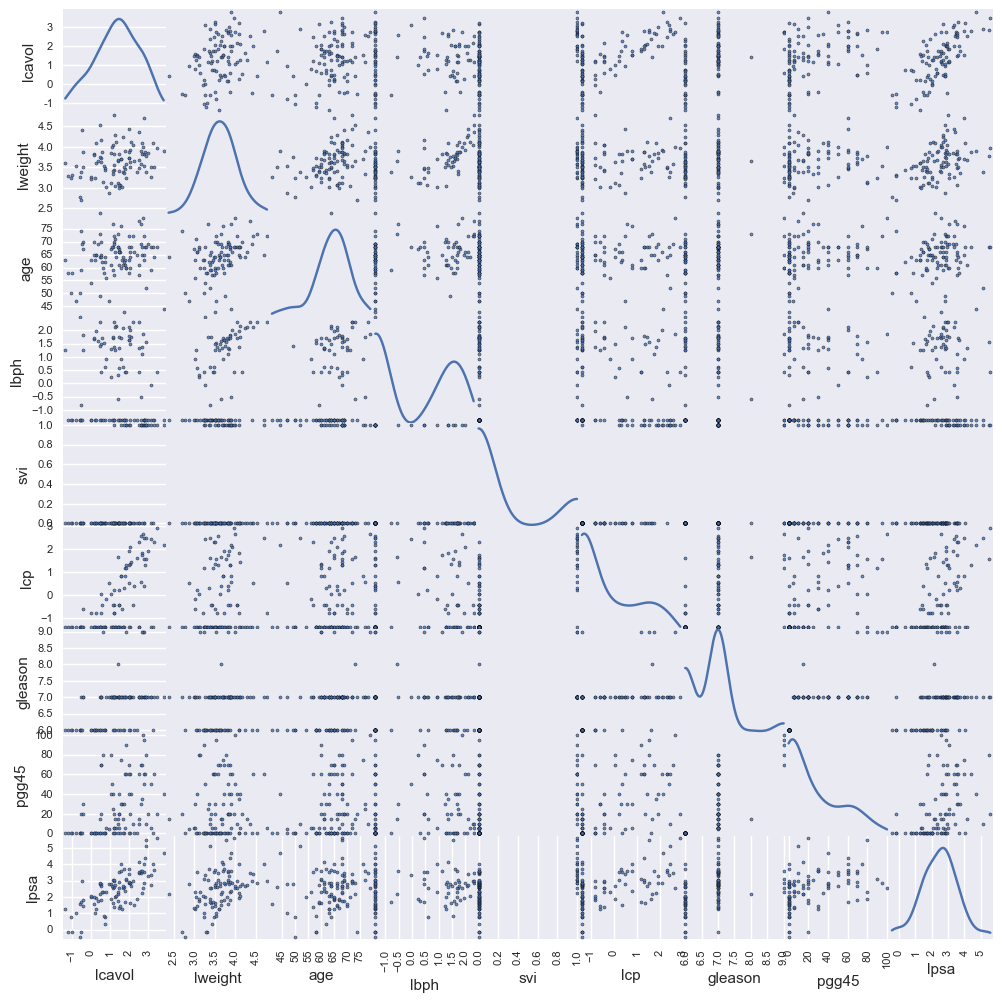In :
aic = lambda rss, n, k: n*np.log(float(rss)/n) + 2*k

In :
from sklearn import linear_model

subsets = [['lcavol', 'lweight', 'age'],
['lweight', 'age', 'lbph'],
['lbph', 'svi', 'lcp'],
['age', 'gleason', 'pgg45'],
['lcavol', 'svi', 'pgg45']]

y = prostate.lpsa

In :
aic_values = []
for i,xvars in enumerate(subsets):

X = prostate[xvars]
regmod = linear_model.LinearRegression()
regmod.fit(X, y)
rss = ((regmod.predict(X) - y) ** 2).sum()

In :
aic_values

Out:
[-56.17644706056128,
12.576322548963725,
-19.645839021059246,
12.788958805386617,
-52.180204316836701]
In :
v = np.exp(-0.5*np.array(aic_values))
aic_weights = v / v.sum()
aic_weights.round(2)

Out:
array([ 0.88,  0.  ,  0.  ,  0.  ,  0.12])
In :
five_fold = cross_validation.KFold(len(y), n_folds=5)
ten_fold = cross_validation.KFold(len(y), n_folds=10)

In :
five_fold_scores = []
for i,xvars in enumerate(subsets):

X = prostate[xvars]
regmod = linear_model.LinearRegression()

scores = cross_validation.cross_val_score(regmod, X, y, scoring='neg_mean_squared_error', cv=five_fold)

five_fold_scores.append(scores.mean())


Note that scikit-learn's MSE is negative.

In :
five_fold_scores

Out:
[-0.89487595018835608,
-1.8409138632046393,
-1.5503052538886308,
-1.8835060063515872,
-0.975753204680327]
In :
ten_fold_scores = []
for i,xvars in enumerate(subsets):

X = prostate[xvars]
regmod = linear_model.LinearRegression()

scores = cross_validation.cross_val_score(regmod, X, y, scoring='neg_mean_squared_error', cv=ten_fold)

ten_fold_scores.append(scores.mean())

In :
ten_fold_scores

Out:
[-0.72880704148344222,
-1.4900220916717231,
-1.1737795318460531,
-1.4894535145600449,
-0.83099392906008041]

The results for each are similar, and it can be shown that AIC is equivalent to a cross-validation outcome. AIC are more easily interpretable, since they can be turned into model probabilities.

In :
X.index.isin(np.random.choice(X.index, len(X)))^True

Out:
array([False,  True, False, False,  True, False,  True, False,  True,
True, False, False, False, False,  True, False, False,  True,
True,  True, False,  True, False, False,  True, False, False,
False, False,  True, False,  True,  True,  True, False,  True,
False, False,  True, False,  True,  True, False, False, False,
False,  True, False,  True, False,  True, False,  True, False,
True,  True, False, False, False, False, False, False,  True,
True, False, False, False, False,  True, False,  True,  True,
False, False, False,  True,  True, False, False, False, False,
False, False, False, False, False, False,  True, False,  True,
False,  True, False, False, False, False, False], dtype=bool)
In :
# Part 2
B = 50
scores_632 = []
for i,xvars in enumerate(subsets):

# Calculate bootstrap sample indices
X = prostate[xvars].values
y_ = y.values
bootstrap_ind = [np.random.choice(range(len(X)), len(X)) for _ in range(B)]

# Fit models to bootstrap samples
regmod = linear_model.LinearRegression()
bootstrap_fits = [regmod.fit(X[i], y_[i]) for i in bootstrap_ind]

# Calculate loss for each observation over models that did not use it to fit the model
loss = [np.mean([(regmod.predict(X[i].reshape(1,-1)) - y_[i])**2 for b in range(B) if i not in bootstrap_ind[b]])
for i in range(len(X))]
# LOO error
err_1 = np.mean(loss)

# Naive (training) error
err_bar = ((regmod.fit(X, y_).predict(X) - y_)**2).mean()

scores_632.append(err_bar)

In :
scores_632

Out:
[0.52676839796284325,
1.0701481958523336,
0.76767401697888016,
1.0724966690506299,
0.54892364856730946]

Note that the above uses MSE and not negative MSE, so the lower values are better here. The results are comparable among the models.

In :
plt.plot(five_fold_scores)
plt.plot(ten_fold_scores)
plt.plot(-np.array(scores_632))

Out:
[<matplotlib.lines.Line2D at 0x12e9ad048>]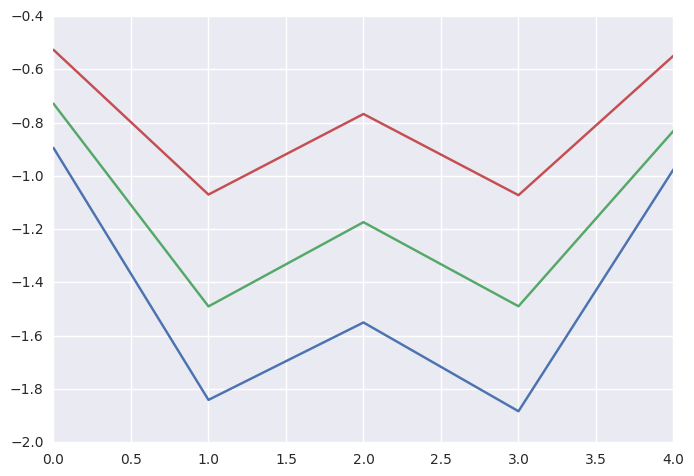Question 4¶

Fit a series of random-forest classifiers to the very low birthweight infant data (vlbw.csv), to explore the sensitivity to the parameter m, the number of variables considered for splitting at each step. Plot both the out-of-bag error as well as the test error against a suitably-chosen range of values for m.

In :
vlbw = pd.read_csv('/Users/fonnescj/Teaching/Bios8366/data/vlbw.csv', index_col=0)
vlbw.ix

Out:
birth             81.511
exit              81.604
hospstay              34
lowph                NaN
pltct                100
race               white
bwt                 1250
gest                  35
inout       born at Duke
twn                    0
lol                  NaN
magsulf              NaN
meth                   0
toc                    0
delivery       abdominal
apg1                   8
vent                   0
pneumo                 0
pda                    0
cld                    0
pvh                  NaN
ivh                  NaN
ipe                  NaN
year              81.512
sex               female
Name: 1, dtype: object
In :
vlbw.ipe.unique()

Out:
array([nan, 'absent', 'definite', 'possible'], dtype=object)
In :
vlbw_numeric = vlbw.replace({'sex': {'female':0, 'male':1},
'delivery': {'abdominal':0, 'vaginal':1},
'inout': {'born at Duke':0, 'transported':1},
'race': {'white':0, 'black':1, 'native American':2, 'oriental':3},
'ivh': {'definite':1, 'absent':0, 'possible':1},
'ipe': {'definite':1, 'absent':0, 'possible':1},
'pvh': {'definite':1, 'absent':0, 'possible':1}})

In :
vlbw_numeric.isnull().mean(0).round(2)

Out:
birth       0.03
exit        0.05
hospstay    0.05
lowph       0.09
pltct       0.10
race        0.04
bwt         0.00
gest        0.01
inout       0.00
twn         0.03
lol         0.57
magsulf     0.37
meth        0.16
toc         0.16
delivery    0.03
apg1        0.05
vent        0.04
pneumo      0.04
pda         0.04
cld         0.10
pvh         0.22
ivh         0.21
ipe         0.21
year        0.03
sex         0.03
dtype: float64

We will drop variables that have a lot of missing values, as well as the response variables

In :
vlbw_numeric = vlbw_numeric.drop(['lol', 'magsulf'], axis=1)

In :
vlbw_complete = vlbw_numeric[vlbw_numeric.ivh.notnull()]
y = vlbw_complete.pop('ivh').values

In :
from sklearn.preprocessing import Imputer

imp = Imputer(missing_values='NaN', strategy='median', axis=0)

In :
imp.fit(vlbw_complete)

Out:
Imputer(axis=0, copy=True, missing_values='NaN', strategy='median', verbose=0)
In :
X = imp.transform(vlbw_complete)

In :
from sklearn.ensemble import RandomForestClassifier

In :
X_train, X_test, y_train, y_test = cross_validation.train_test_split(
X, y, test_size=0.4, random_state=0)

In :
m = [1, 3, 5, 10, 15, 20]

oob_score = []
test_score = []
for mi in m:

rfc = RandomForestClassifier(max_features=mi)
rfc.fit(X_train, y_train)
test_score.append(rfc.score(X_test, y_test))

rfc = RandomForestClassifier(max_features=mi, oob_score=True)
rfc.fit(X_train, y_train)
oob_score.append(rfc.score(X_test, y_test))

/Users/fonnescj/anaconda3/envs/bios8366/lib/python3.5/site-packages/sklearn/ensemble/forest.py:439: UserWarning: Some inputs do not have OOB scores. This probably means too few trees were used to compute any reliable oob estimates.
warn("Some inputs do not have OOB scores. "
/Users/fonnescj/anaconda3/envs/bios8366/lib/python3.5/site-packages/sklearn/ensemble/forest.py:439: UserWarning: Some inputs do not have OOB scores. This probably means too few trees were used to compute any reliable oob estimates.
warn("Some inputs do not have OOB scores. "
/Users/fonnescj/anaconda3/envs/bios8366/lib/python3.5/site-packages/sklearn/ensemble/forest.py:439: UserWarning: Some inputs do not have OOB scores. This probably means too few trees were used to compute any reliable oob estimates.
warn("Some inputs do not have OOB scores. "
/Users/fonnescj/anaconda3/envs/bios8366/lib/python3.5/site-packages/sklearn/ensemble/forest.py:439: UserWarning: Some inputs do not have OOB scores. This probably means too few trees were used to compute any reliable oob estimates.
warn("Some inputs do not have OOB scores. "
/Users/fonnescj/anaconda3/envs/bios8366/lib/python3.5/site-packages/sklearn/ensemble/forest.py:439: UserWarning: Some inputs do not have OOB scores. This probably means too few trees were used to compute any reliable oob estimates.
warn("Some inputs do not have OOB scores. "
/Users/fonnescj/anaconda3/envs/bios8366/lib/python3.5/site-packages/sklearn/ensemble/forest.py:439: UserWarning: Some inputs do not have OOB scores. This probably means too few trees were used to compute any reliable oob estimates.
warn("Some inputs do not have OOB scores. "

In :
plt.plot(m, oob_score)
plt.plot(m, test_score)

Out:
[<matplotlib.lines.Line2D at 0x12e95d5f8>]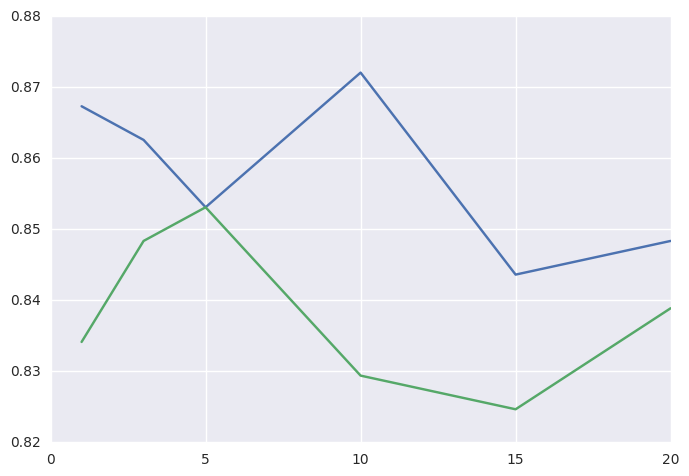Bonus: Question 5¶

Use a grid search to optimize the number of estimators and max_depth for a Gradient Boosted Decision tree using the very low birthweight infant data. Plug this optimal max_depth into a single decision tree. Does this single tree over-fit or under-fit the data? Repeat this for the Random Forest. Construct a single decision tree using the max_depth which is optimal for the Random Forest. Does this single tree over-fit or under-fit the data?

In :
from sklearn import grid_search

parameters = {'max_depth': [1, 2, 3, 5, 7], 'n_estimators': [25, 50, 100, 150]}
gbc_cv = grid_search.GridSearchCV(gbc, parameters)

In :
gbc_cv.fit(X, y)

Out:
GridSearchCV(cv=None, error_score='raise',
learning_rate=0.1, loss='deviance', max_depth=3,
max_features=None, max_leaf_nodes=None,
min_impurity_split=1e-07, min_samples_leaf=1,
min_samples_split=2, min_weight_fraction_leaf=0.0,
n_estimators=100, presort='auto', random_state=None,
subsample=1.0, verbose=0, warm_start=False),
fit_params={}, iid=True, n_jobs=1,
param_grid={'n_estimators': [25, 50, 100, 150], 'max_depth': [1, 2, 3, 5, 7]},
pre_dispatch='2*n_jobs', refit=True, scoring=None, verbose=0)
In :
best_gbc_params = gbc_cv.best_params_

In :
gbc_single = GradientBoostingClassifier(max_depth=best_gbc_params['max_depth'],
n_estimators=best_gbc_params['n_estimators'])
gbc_single.fit(X_train, y_train)
gbc_single.score(X_test, y_test)

Out:
0.86729857819905209
In :
gbc_single.score(X_train, y_train)

Out:
0.84810126582278478
In :
rfc = RandomForestClassifier()
rf_cv = grid_search.GridSearchCV(rfc, parameters)

In :
rf_cv.fit(X, y)

Out:
GridSearchCV(cv=None, error_score='raise',
estimator=RandomForestClassifier(bootstrap=True, class_weight=None, criterion='gini',
max_depth=None, max_features='auto', max_leaf_nodes=None,
min_impurity_split=1e-07, min_samples_leaf=1,
min_samples_split=2, min_weight_fraction_leaf=0.0,
n_estimators=10, n_jobs=1, oob_score=False, random_state=None,
verbose=0, warm_start=False),
fit_params={}, iid=True, n_jobs=1,
param_grid={'n_estimators': [25, 50, 100, 150], 'max_depth': [1, 2, 3, 5, 7]},
pre_dispatch='2*n_jobs', refit=True, scoring=None, verbose=0)
In :
best_rf_params = rf_cv.best_params_

In :
from sklearn import tree

tree_classsifier = tree.DecisionTreeClassifier(max_depth=best_rf_params['max_depth'])
tree_classsifier.fit(X_train, y_train)
tree_classsifier.score(X_test, y_test)

Out:
0.80568720379146919
In :
tree_classsifier.score(X_train, y_train)

Out:
0.879746835443038